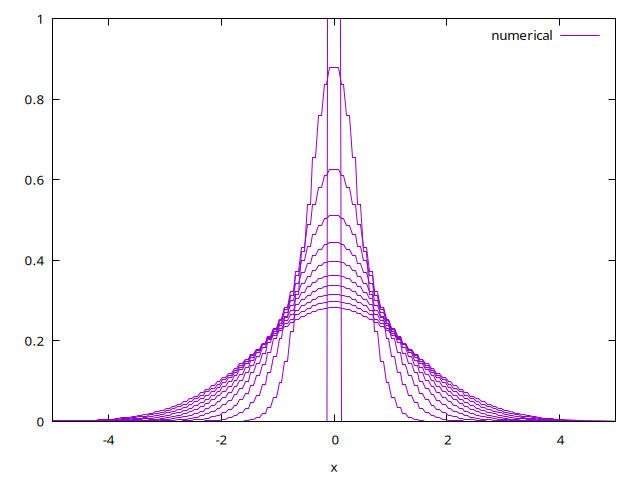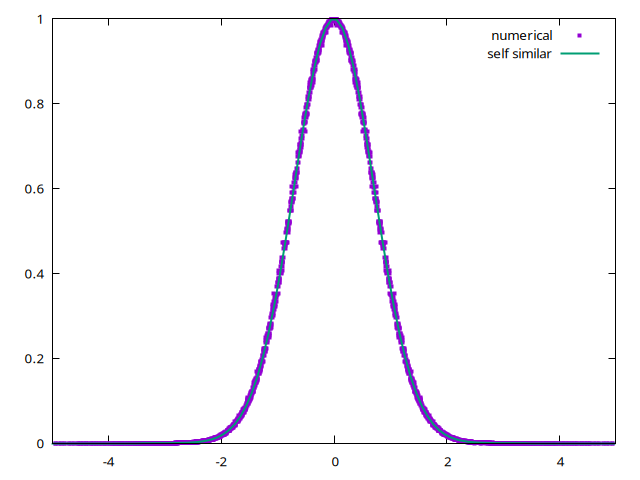# Resolution of heat equation

Explicit resolution of the heat equation \displaystyle \frac{\partial T}{\partial t}= \frac{\partial^2 T}{\partial x^2} with a given total energy at initial time \int_{-\infty}^{\infty}Tdx=1.

with finite volumes: \displaystyle \frac{T_i^{n+1}- T_i^{n}}{\Delta t } = -\frac{q_{i+1} - q_i}{\Delta}
set size ratio .5
set samples 9
set label "T i-1" at 1.5,3.1
set label "T i" at 2.5,3.15
set label "T i+1" at 3.5,2.5
set xtics ("i-2" 0.5, "i-1" 1.5, "i" 2.5,"i+1" 3.5,"i+2" 4.5,"i+3" 5.5)
set arrow from 2,1 to 2.5,1
set arrow from 3,1 to 3.5,1
set label "q i" at 2.1,1.25
set label "q i+1" at 3.1,1.25

set label "x i-1/2" at 1.5,0.25
set label "x i" at 2.4,0.25
set label "x i+1/2" at 3.,0.25

set label "x"  at 0.5,2+sin(0)
set label "x"  at 1.5,2+sin(1)
set label "x"  at 2.5,2+sin(2)
set label "x"  at 3.5,2+sin(3)
set label "x"  at 4.5,2+sin(4)
set label "x"  at 5.5,2+sin(5)
p[-1:7][0:4] 2+sin(x) w steps not,2+sin(x) w impulse not linec 1(script)

#include "grid/cartesian1D.h"
#include "run.h"

scalar T[];
double dt;

int main() {
L0 = 10.;
X0 = -L0/2;
N = 200;
DT = (L0/N)*(L0/N)/2 ;
#define EPS 0.1
run();
}

initial temperature a “Dirac”, in fact a thin rectangle so that \int_{-\infty}^{\infty}Tdx=1.

event init (t = 0) {
foreach()
T[] =  1./EPS*(fabs(x)<EPS)/2;
boundary ({T});
}

print data

event printdata (t += 0.1; t < 1) {
foreach()
fprintf (stdout, "%g %g %g\n", x, T[], t);
fprintf (stdout, "\n\n");
}

integration

event integration (i++) {
double dt = DT;
scalar dT[],q[];

finding the good next time step

  dt = dtnext (dt);

explicit step, the well known approximation of the second order derivative \displaystyle \frac{ T(x+\Delta x) - 2 T(x) +T(x-\Delta x)}{\Delta x^2 } \simeq \frac{\partial^2 T}{\partial x^2} is as well a flux divergence: -\frac{\partial q }{\partial x} with a
discrete flux across interface between ì-1 and ì: q_i = - \frac{ T_{i} - T_{i-1}}{\Delta }

  foreach()
q[]=-(T[0,0] - T[-1,0])/Delta;
boundary ({q});

and then \frac{\partial T}{\partial t}= -\frac{\partial q }{\partial x} is the balance between flux leaving q_{i+1} and flux entering q_i the cell \displaystyle T_i^{n+1}= T_i^{n } - (\Delta t ) ( q_{i+1} - q_i)/\Delta

  foreach()
dT[] = - ( q[1,0]  - q[0,0] )/Delta;

update

  foreach()
T[] += dt*dT[];
boundary ({T});
}

# Run

 ln -s ../../Makefile Makefile
make heat.tst;make heat/plots
make heat.c.html ; open heat.c.html 

or with make

 make heat.tst;make heat/plots
make heat.c.html ; open heat.c.html 

# Results

After running, it gives the gaussian decrease:

reset
set xlabel "x"
set output 'plot1.png'
p[-5:5][:1]'out' u ($1):($2) t 'numerical' w l(script)

The self similar analytical solution is \displaystyle \theta = \frac{1}{2 \sqrt{\pi t} } e^{-x^2/4 t}

the solution can be written in self similar variables: we plot  as a function of x/\sqrt(4t) and superpose e^{-x^2}

reset
set output 'plot.png'
p[-5:5][:1]'out' u ($1/sqrt(4*$3)):($2*sqrt($3*pi)*2) t 'numerical' w p pt 5 ps 0.5, exp(-x*x) t 'self similar' lw 2(script)

heat.c the explicit heat equation

heat_imp.c the implicit heat equation

# Exercise

Change DT to test the stablity

Change the Initial temperature to test the erf case

# Bibliography

ready for new site 09/05/19